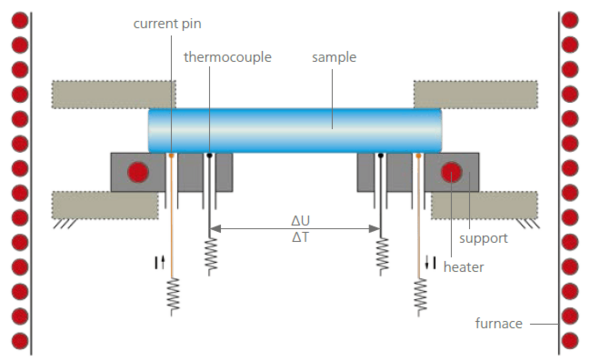Glossary

# Electrical Conductivity (SBA)

Electrical conductivity is a physical property indicating a material's ability to allow the transport of an electric charge. The SI unit is Siemens per meter [S/m], named after Werner von Siemens. It is commonly represented by the Greek letter σ (sigma), κ (kappa) or γ (gamma).

Electrical conductivity is defined as a proportionality factor between the current DensityThe mass density is defined as the ratio between mass and volume. density and the electrical field strength. In a special case of constant electrical conductivity, this definition conforms to Ohm's law.

Electrical conductivity is the reciprocal value of the Electrical ResistivityElectrical resistivity or electrical resistance is a fundamental material property that quantifies how strongly a given material opposes the flow of electric current.electrical resistivity.

A conductor such as a metal has high conductivity, and an insulator like glass or a vacuum has low conductivity. A semiconductor has a conductivity that varies widely under different conditions.

A well-known technique for determining electrical conductivity is the four-point probe method, which can be carried out with the NETZSCH Seebeck-Analyzer (NETZSCH SBA 458 Nemesis®®). Both properties – the electrical conductivity and the Seebeck coefficient – can be measured simultaneously in the temperature range from -125°C to 800°C or 1100°C, depending on the measuring head used.Measurement setup of the SBA 458 Nemesis®® for the temperature range between RT and 1100°C